# RD Sharma Solutions for Class 12 Maths Exercise 19.32 Chapter 19 Indefinite Integrals

RD Sharma Solutions for Class 12 Maths Exercise 19.32 Chapter 19 Indefinite Integrals is provided here. Integration of some special irrational algebraic functions is the main topic covered in this exercise. The solutions for RD Sharma book, solved by BYJU’S experts, explain the steps most easily without missing out on essential aspects of solving a question.

Students can easily view and download the RD Sharma Solutions for Class 12 Exercise 19.32 of Chapter 19 Indefinite Integrals, from the below-provided links. These RD Sharma Solutions primarily improve their speed in solving problems, which builds time management, which is key from the exam perspective.

## RD Sharma Solutions for Class 12 Chapter 19 – Indefinite Integrals Exercise 19.32

### Exercise 19.32 Page No: 19.196

Evaluate the following integrals: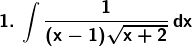Solution: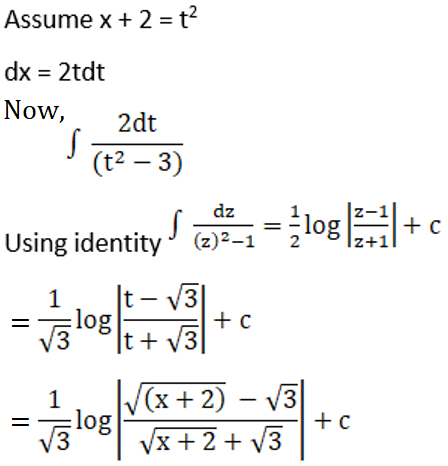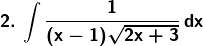Solution: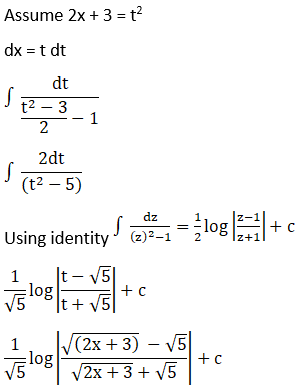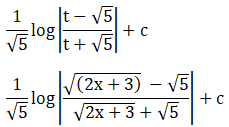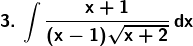Solution: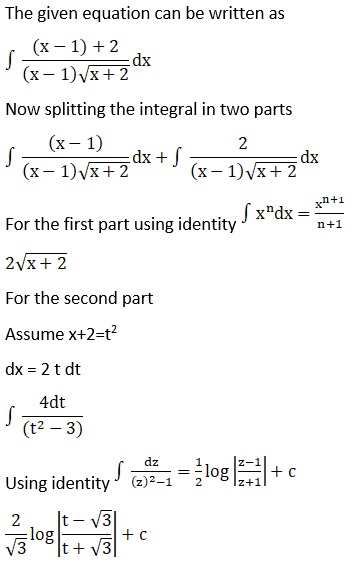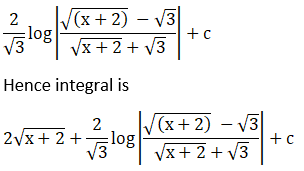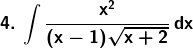Solution: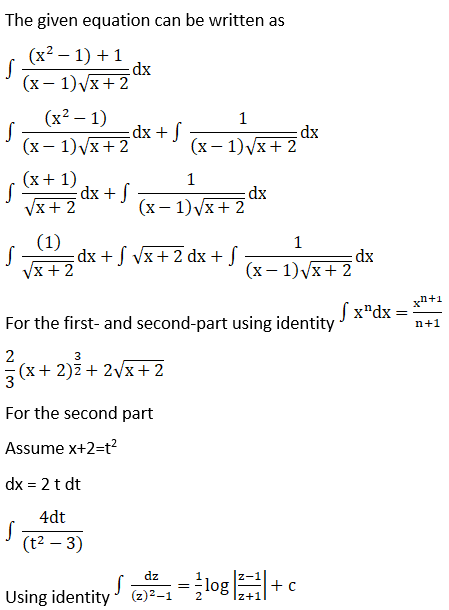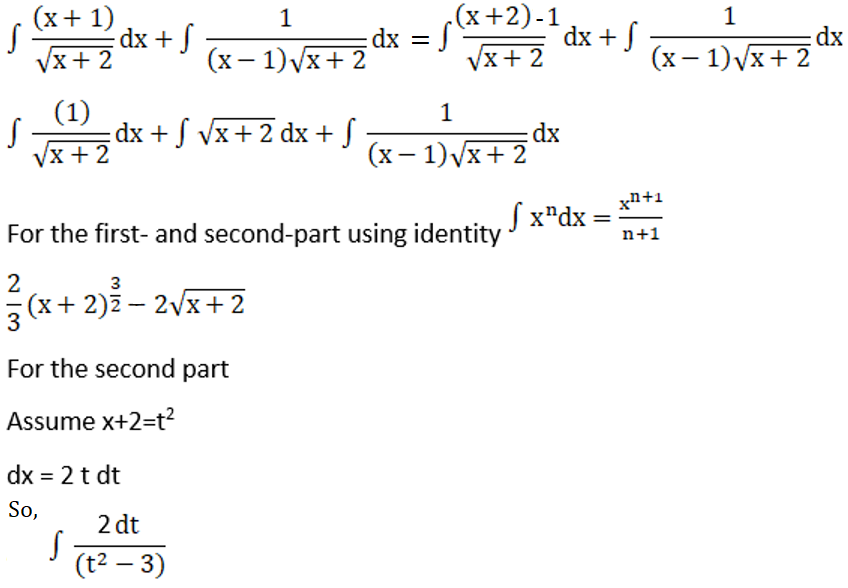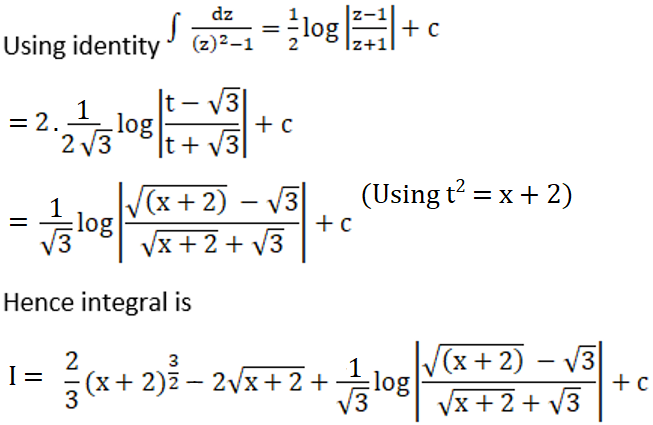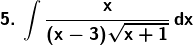Solution: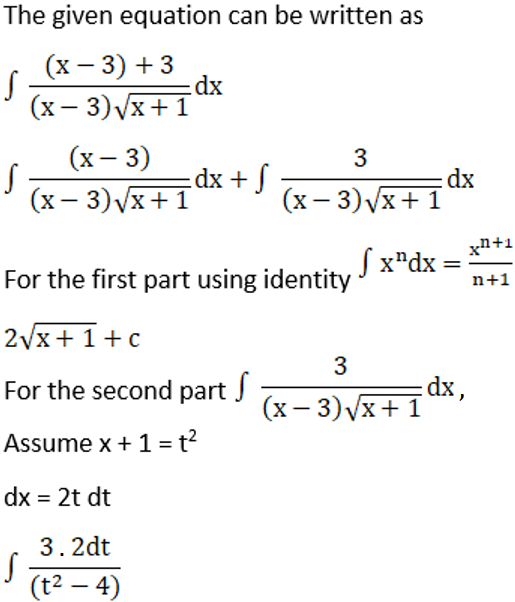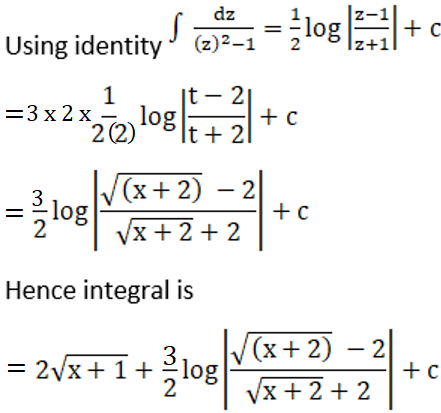### Access other exercises of RD Sharma Solutions for Class 12 Chapter 19 – Indefinite Integrals

Exercise 19.1 Solutions

Exercise 19.2 Solutions

Exercise 19.3 Solutions

Exercise 19.4 Solutions

Exercise 19.5 Solutions

Exercise 19.6 Solutions

Exercise 19.7 Solutions

Exercise 19.8 Solutions

Exercise 19.9 Solutions

Exercise 19.10 Solutions

Exercise 19.11 Solutions

Exercise 19.12 Solutions

Exercise 19.13 Solutions

Exercise 19.14 Solutions

Exercise 19.15 Solutions

Exercise 19.16 Solutions

Exercise 19.17 Solutions

Exercise 19.18 Solutions

Exercise 19.19 Solutions

Exercise 19.20 Solutions

Exercise 19.21 Solutions

Exercise 19.22 Solutions

Exercise 19.23 Solutions

Exercise 19.24 Solutions

Exercise 19.25 Solutions

Exercise 19.26 Solutions

Exercise 19.27 Solutions

Exercise 19.28 Solutions

Exercise 19.29 Solutions

Exercise 19.30 Solutions

Exercise 19.31 Solutions